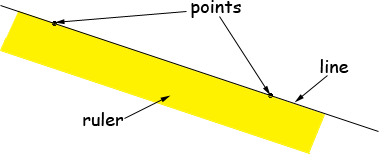Name: Crystal Who is asking: Student Level of the question: Middle Question: How do you graph 3x + 6y = -9 by axis intercepts? Hi Crystal, The first thing to realize is that the graph of ax + by = c, where a, b and c are numbers, is a straight line. A nice property of a straight line is that if you know any two points on the line you can plot the entire line. Just put your ruler down so that both points are at the edge of the ruler and then draw the line.The easiest two points to choose are the intercepts. The y-intercept occurs when x = 0 and the x-intercept occurs when y = 0. In your equation the y-intercept occurs when x = 0, that is 3(0) + 6y = -9 so y = -9/6 = -3/2 and the x-intercept occurs when y = 0, that is 3x + 6(0) = -9 so x =  -9/3 = -3 Thus the intercepts are (0, -3/2) and (-3,0). Plot the points and draw the line, Penny